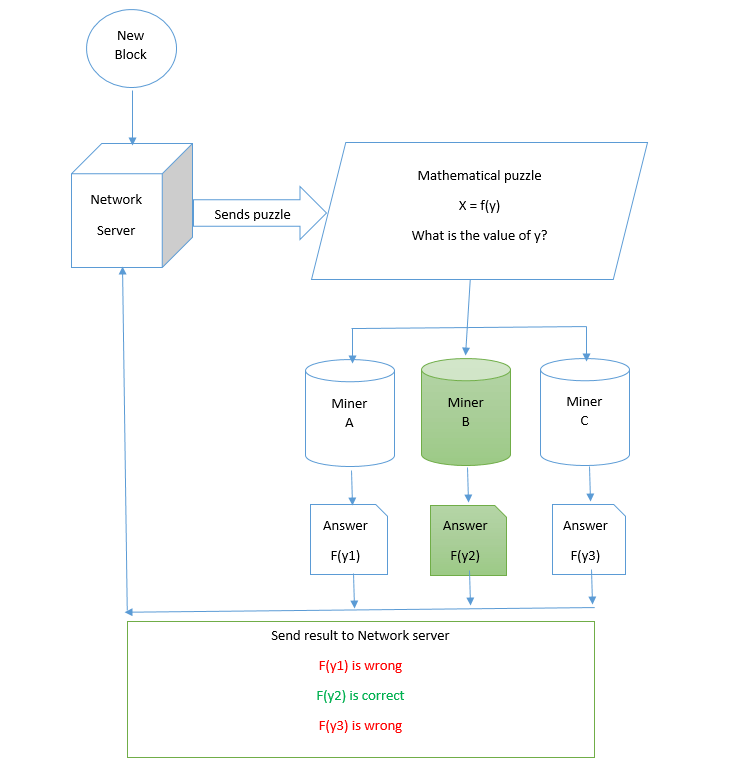# Blockchain Consensus Algorithm: Proof of Stake Vs. Proof of WorkA consensus algorithm is a process used to achieve agreement on a single data which are designed for creating reliability in a network involving multiple nodes. In view of many Blockchain Consulting companies, it is helpful algorithm for solving the issues in distributed computing and Multi agent systems.

There are multiple kinds of consensus algorithms in Blockchain Technology which currently exists. Let’s know the difference between two of them Proof of Stake and Proof of Work.

## Proof of Stake (PoS):

Proof of Stake (PoS) is a mining algorithm that is based on the stake of the miner in the given ecosystem unlike arithmetic difficult puzzle in Proof of Work (PoW). That means, who writes the next block is decided based on how large the stake of a person / miner holds (i.e. how many coins or tokens does it hold).

For example,

Miner 1 – stakes 50 coins (bitcoin or any other)

Miner 2 – stakes 25 coins

Miner 3 – stakes 10 coins

Miner 4 – stakes 75 coins

Miner 4 would be given opportunity to write and validate the next block. Miner 4 receives transaction fees unlike block reward in Proof of Work (PoW).

• This does not require high computing power to reach a consensus and thus energy saving is very high and thus the solutions are energy efficient.

## References:

https://www.coindesk.com/short-guide-blockchain-consensus-protocols/

## Proof of Work (PoW):

Proof of Work is consensus algorithm that decides which node writes the new block from the given network. Proof of Work is followed by Bitcoin and Ethereum both with minor variation. With PoW, each minor or node competes with each other and gets rewarded at the end. Each node/miner is given common mathematical puzzle or function to solve. The mathematical puzzle is normally complex and takes time to solve the same. Whichever miner solves the puzzle, gets an opportunity to write the new block to the chain.Mathematical puzzle is normally a puzzle that requires a lot of computational energy to solve the same. The answer of the PoW problem or mathematical equation is called hash . Let us understand in above diagram.

We have set of new transactions to add to chain. In current system, we have three nodes (miner) – A, B and C. Network server sends mathematical puzzle to all three. The mathematical puzzle is solved by all three miners and response is sent to Network server. Network server finds that miner B has solved the puzzle correctly and thus Miner B writes and confirms the transaction entry to the chain.

## Example of mathematical problem:

Find a number n such that its hash value of n appended to X gives a HASH that starts with 0.

X = “block”;

Hash(X) = Hash(block) = 496aca80e4d8f29fb8e8cd816c3afb48d3f103970b3a2ee1600c08ca67326dee

Hash(X1) = Hash(block1) = 9a59c5f8229aab55e9f855173ef94485aab8497eea0588f365c871d6d0561722

.

.

Hash(X4) = Hash(block4) = 215008ba416eb06b8cfd53814660a43255e4ccc8703080af501ea0eaf7b7fdea

Result n is 4. Miner sends 4 to Network server and Network server validates the result. If correct, miner writers and confirms the block.

## Few challenges of Proof of Work:

High energy consumption – PoW requires hashing millions of values every minute to get the desired result. This consumes lot of energy and power. Bitcoin miners use specific chips designed specifically for hashing. The current hash rate of bitcoin is around 20 hash per second i.e. Approximately 20 billion hashes per second.

Miner centralization – Due to specific hardware requirements, most of the miners are currently based in China as it offers high speed chips that are required for good mining.